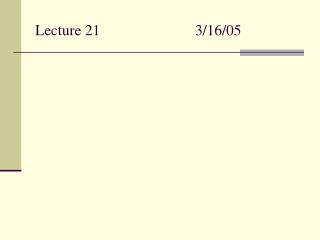# Lecture 21 3/16/05 - PowerPoint PPT PresentationDownload PresentationLecture 21 3/16/05

Lecture 21 3/16/05
Download Presentation## Lecture 21 3/16/05

- - - - - - - - - - - - - - - - - - - - - - - - - - - E N D - - - - - - - - - - - - - - - - - - - - - - - - - - -
##### Presentation Transcript

1. Lecture 21 3/16/05

2. Quiz 3/18/05 1) For the titration of 100 mL of 0.15 M HCl with 0.1 M NaOH, fill in the table. 2) Why does adding water to a buffer solution not change the pH?

3. Give the identity and concentration of the titrated sample (50 mL) represented by the blue line.

4. Problem 98 • Describe the effect on the pH of • Adding sodium acetate to 0.1 M acetic acid • Adding NaNO3 to 0.1 M HNO3 • Why are the effects not the same?

5. In lab, you titrate a 25-mL sample of HCN with 0.075 M NaOH. If it takes 37.5 mL to reach the equivalence point, what is the concentration of the HCN?

6. Which of these combinations would be the best to buffer the pH at approximately 9? • CH3COOH (Ka = 1.8 x 10-5) / NaCH3COO • HCl (Ka = very large) / HCl • NH3 (Kb = 1.8 x 10-5) / NH4+

7. Each of the solutions given in the table has an equal volume and the same concentration (0.1 M) Which solution requires the greatest volume of 0.1 M NaOH to titrate? Explain.

8. The solubility of Ca(OH)2 is 7.9 x 10-6. What is the pH of a saturated solution of calcium hydroxide?

9. A 25-mL sample of 0.085 M pyridine (Kb = 1.5 x 10-9) is titrated with 0.102 M HCl. What is the pH when 5.5 mL of the acid has been added?